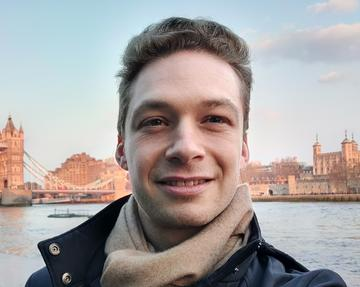# Emilio Rossi-Ferrucci### Abstract

We apply the rough-Skorokhod integral conversion formula of [Cass Lim 2020] to the RDE for the signature of certain Gaussian processes of bounded 4>p-variation, which include fractional Brownian motion (fBm) with Hurst parameter H>1/4. This results in a recursive formula relating each level of the signature to Skorokhod and Young integrals against the variance and covariance functions of the signature terms of lower order and their products. We are then able to derive a non-recursive formula, which expresses the signature as a sum of mixed Skorokhod/Young iterated integrals. Next, we show how to pass to the expectation in this identity, and express the expected signature in closed form as a sum of iterated integrals against the variance and covariance functions. Our mixed iterated integrals are represented using diagrams which play a role similar to that of rooted forests in branched rough path theory, and the operation of taking the expectation is given a simple combinatorial description in terms of such diagrams. When p<2 these calculations simplify somewhat, and we reobtain the expression for the expected signature of 1/2<H-fBm proved in [Baudoin Coutin 2007]. This is joint work with Thomas Cass.